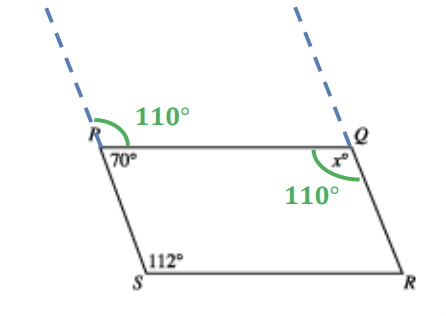# ACT: Deep Dive into Mathematics Quiz

This is an MCQ-based quiz on the topic of ACT: Deep Dive into Mathematics.

This set of MCQs helps you brush up on the important mathematics topic and prepare you to dive into skill practice and expand your knowledge to tackle the question and answer it carefully.

Start Quiz

In quadrilateral PQRS below, sides PS and QR are parallel for what value of x ?160

110

100

120

A typical high school student consumes 67.5 pounds of sugar per year. As part of a new nutrition plan, each member of a track team plans to lower the sugar he or she consumes by at least 20% for the coming year. Assuming each track member had consumed sugar at the level of a typical high school student and will adhere to this plan for the coming year, what is the maximum number of pounds of sugar to be consumed by each track team member in the coming year?

14

44

48

54

How many irrational numbers are there between 1 and 6 ?

1

3

4

Infinte

The length, in inches, of a box is 3 inches less than twice its width, in inches. Which of the following gives the length, l inches, in terms of the width, w inches, of the box?

i = w + 3

i = w - 3

i = 2w - 3

i = 2w + 3

Sales for a business were 3 million dollars more the second year than the first, and sales for the third year were double the sales for the second year. If sales for the third year were 38 million dollars, what were sales, in millions of dollars, for the first year?

16

17.5

20.5

22

What is the greatest common factor of 42, 126, and 210 ?

2

6

42

21

What is the greatest common factor of 42, 126, and 210 ?

2

6

42

21

What is the value of x when 2x + 3 = 3x – 4 ?

-7

-1/5

1

7

When x = 3 and y = 5, by how much does the value of 3x^2 – 2y exceed the value of 2x^2 – 3y ?

4

14

16

20

Abandoned mines are frequently filled with water. Before an abandoned mine can be reopened, the water must be pumped out. The size of the pump required depends on the depth of the mine. If pumping out a mine that is D feet deep requires a pump that pumps a minimum of  4250 gallons per minute, pumping out a mine that is 150 feet deep would require a pump that pumps a minimum of how many gallons per minute?

362

500

800

1250

Quiz/Test Summary
Title: ACT: Deep Dive into Mathematics Quiz
Questions: 10
Contributed by: